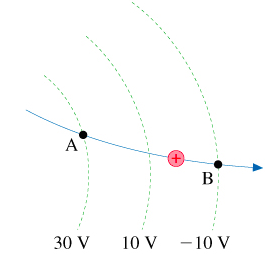# Problem: A proton's speed as it passes point A is 50000 m/s . It follows the trajectory shown in the figure.(Figure 1)What is the proton's speed at point B?

###### FREE Expert Solution

We'll use the law of conservation of energy:

$\overline{){\mathbf{P}}{\mathbf{.}}{{\mathbf{E}}}_{{\mathbf{i}}}{\mathbf{+}}{\mathbf{K}}{\mathbf{.}}{{\mathbf{E}}}_{{\mathbf{i}}}{\mathbf{=}}{\mathbf{P}}{\mathbf{.}}{{\mathbf{E}}}_{{\mathbf{f}}}{\mathbf{+}}{\mathbf{K}}{\mathbf{.}}{{\mathbf{E}}}_{{\mathbf{f}}}}$

The work done by a proton is given by:

$\begin{array}{rcl}\mathbf{W}& \mathbf{=}& \mathbf{q}\mathbf{V}\\ & \mathbf{=}& \mathbf{\left(}\mathbf{1}\mathbf{.}\mathbf{602}\mathbf{×}{\mathbf{10}}^{\mathbf{-}\mathbf{19}}\mathbf{\right)}\mathbf{\left[}\mathbf{30}\mathbf{-}\mathbf{\left(}\mathbf{-}\mathbf{10}\mathbf{\right)}\mathbf{\right]}\end{array}$

W = 6.408 × 10-18 J

95% (42 ratings)###### Problem Details

A proton's speed as it passes point A is 50000 m/s . It follows the trajectory shown in the figure.(Figure 1)What is the proton's speed at point B?